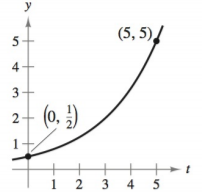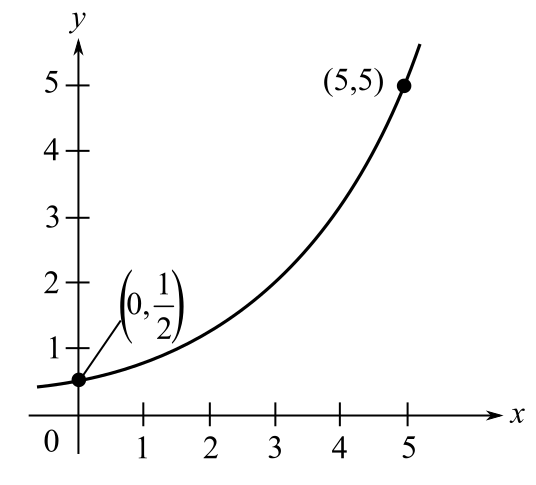Chapter 4.6, Problem 2E### Calculus: An Applied Approach (Min...

10th Edition
Ron Larson
ISBN: 9781305860919

#### Solutions

Chapter
Section### Calculus: An Applied Approach (Min...

10th Edition
Ron Larson
ISBN: 9781305860919
Textbook Problem
1 views

# Modeling Exponential Growth and Decay In Exercises 1-6, find the exponential function y = C e k t that passes through the two given points. y = C e k tTo determine

To calculate: The exponential function y=Cekt for the following graph:Explanation

Given Information:

The provided graph is:

Calculation:

Consider the following graph:

Observe that the exponential function curve passes through the points (0,12) and (5,5).

Hence the points (0,12) and (5,5) satisfy the equation of exponential function y=Cekt.

Substitute t=0,y=12 in the equation y=Cekt.

12=Cek(0)12=Ce012=C1C=12

Hence, the value of C is 12.

Substitute t=5,y=5 in the equation y=12ekt.

5=12ek(5)5=12e5k

Solve the equation 5=12e5k for k

### Still sussing out bartleby?

Check out a sample textbook solution.

See a sample solution

#### The Solution to Your Study Problems

Bartleby provides explanations to thousands of textbook problems written by our experts, many with advanced degrees!

Get Started

#### Solve the inequality. 49. |x 4| 1

Single Variable Calculus: Early Transcendentals, Volume I

#### Find the median for the following set of scores: 1, 9, 3, 6, 4, 3, 11, 10

Essentials of Statistics for The Behavioral Sciences (MindTap Course List)

#### Find y and y. y = ln(l + ln x)

Single Variable Calculus: Early Transcendentals